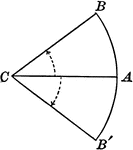### Positive and Negative Arcs in Circles

Illustration showing arcs measured in positive and negative angles.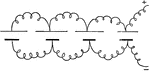### Parallel Connection

"When all of the positive plates are connected on one side, and all of the negative plates are connected…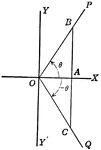### Coordinate Axis With Perpendiculars Drawn To Form Similar Right Triangles From Positive and Negative Theta, Θ

Angle XOP=Θ and angle XOQ=- Θ. From a point in the terminal side of each a perpendicular line is drawn…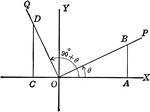### Coordinate Axis With Perpendiculars Drawn To Form Similar Right Triangles

Angle XOP=Θ and angle XOQ=90+Θ. From a point in the terminal side of each a perpendicular line is…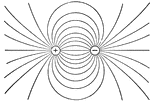### Electricity

This illustration shows the field between two equally and oppositely charged spheres, mapped out by…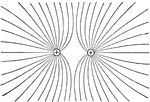### Electricity

This illustration represents the field between two equal positive charges; in this case the lines of…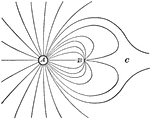### Electricity

This illustration shows the lines of force when a positive charge equal to 4 at A, and a negative charge…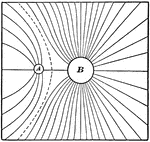### Electricity

This illustration shows the field of force due to a charge 1 at A, and a like charge 4 at B.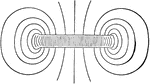### Electricity

This illustration shows the field of force between two parallel planes. At the edges its lines of foce…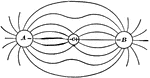### Electricity

A is a positively charged conductor and B represents the equal and oppsite charge. When the conductor…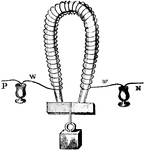### Magnet

"The coils of wire may begin near one pole of the magnet and terminate near the other, or the wire may…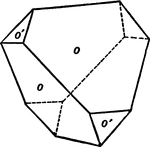### Positive and Negative Tetrahedron in Combination

This form shows a positive and negative tetrahedron in combination.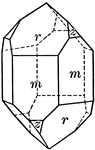### Quartz

"Terminated usually by a combination of a positive and negative rhombohedron, which often are so equally…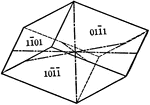### Negative rhombohedron

"The rhombohedron is a form consists of six rhombic-shaped faces, which correspond in their position…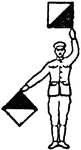### Signal K

Signal for negative and the letter K.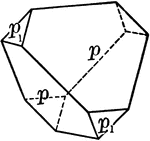### Sphenoid, positive and negative

"It consists of four isoceles triangular faces which intersect all three of the crystallographic axes,…### Negative tetrahedron

"The tetrahedron is a form composed of four equilateral triangular faces, each of which intersects all…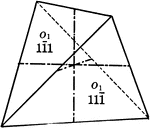### Positive and negative tetrahedrons

"If a positive and negative tetrahedron occured together with equal development, the resulting crystal…